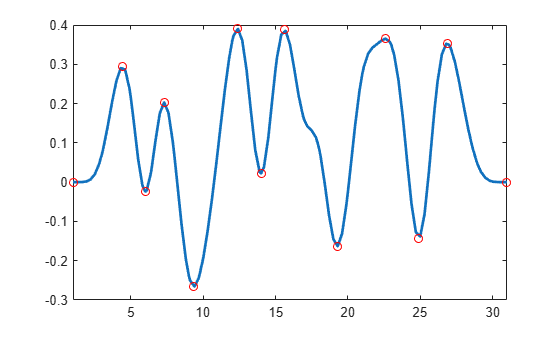Main Content

# fnzeros

Roots of spline

## Syntax

``x = fnzeros(spline)``
``x = fnzeros(spline,I)``

## Description

example

````x = fnzeros(spline)` finds the zeros of a univariate spline in its basic interval. A spline zero is either a point or a closed interval over which the spline is zero.```
````x = fnzeros(spline,I)` finds the zeros in the interval `I`.```

## Examples

collapse all

Create and plot a piecewise linear spline.

```spline = spmak(augknt(1:7,2),[1,0,1,-1,0,0,1]); figure fnplt(spline)```Find the roots of the spline. The spline has each of the three kinds of zeros: touch zero, cross zero, and zero over an interval.

`x = fnzeros(spline)`
```x = 2×3 2.0000 3.5000 5.0000 2.0000 3.5000 6.0000 ```

Plot the roots of the spline using right-pointing triangles at the left endpoints and left-pointing triangles at the right endpoints.

```nz = size(x,2); hold on plot(x(1,:),zeros(1,nz),'r>',x(2,:),zeros(1,nz),'r<','MarkerSize',10) hold off```Create a spline with many local extrema. Locate the local extrema of the spline by finding the roots of its first derivative.

```spline = spmak(1:31,rand(1,25)-0.5); x = fnzeros(fnder(spline));```

Plot the spline and its local extrema.

```figure fnplt(spline) hold on x = unique(x(:)); y = fnval(spline,x); plot(x,y,'ro') xlim([-Inf Inf]) hold off```Create and plot a discontinuous piecewise linear spline.

``` spline = spmak([0 0 1 1 2 2],[-1 1 -1 1]); figure fnplt(spline);```Find the roots of the spline. The roots include the jump through zero at x = 1.

`x = fnzeros(spline)`
```x = 2×3 0.5000 1.0000 1.5000 0.5000 1.0000 1.5000 ```

## Input Arguments

collapse all

Spline, specified as a structure.

Data Types: `struct`

Interval to find zeros in, specified as a numeric vector with two elements.

## Output Arguments

collapse all

Locations of roots of spline, returned as a matrix with two rows. The elements of the first row are the left endpoints of the intervals and the elements of the second row are the right endpoints. Each column contains the left and right endpoint of a single interval. The roots are in increasing order.

There are three different types of intervals:

• If the endpoints are different, then the spline is zero on the entire interval. In this case, the largest possible interval is given, regardless of knots that may be in the interior of the interval.

• If the endpoints are the same and coincident with a knot, then the spline has a zero at that point. The spline could cross zero, touch zero, or be discontinuous at this point.

• If the endpoints are the same and not coincident with a knot, then the spline has a zero crossing at this point.

## Algorithms

To find the roots of a spline, `fnzeros` first converts the spline to B-form. The function then performs some preprocessing to handle discontinuities and then uses the algorithm of .

 Mørken, Knut, and Martin Reimers. "An unconditionally convergent method for computing zeros of splines and polynomials." Mathematics of Computation 76, no. 258 (2007): 845-865.

## Support

#### Machine Learning Challenges: Choosing the Best Classification Model and Avoiding Overfitting

Download white paper